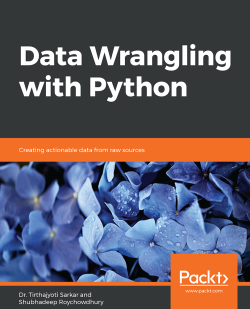### Data Wrangling with Python4.3 (3 reviews total)
By Dr. Tirthajyoti Sarkar , Shubhadeep Roychowdhury
• FREE Subscribe Access now
• \$39.99 Print + eBook
• \$31.99 eBook
• Constantly updated with 100+ new titles each month
• Breadth and depth in over 1,000+ technologies

For data to be useful and meaningful, it must be curated and refined. Data Wrangling with Python teaches you the core ideas behind these processes and equips you with knowledge of the most popular tools and techniques in the domain.

The book starts with the absolute basics of Python, focusing mainly on data structures. It then delves into the fundamental tools of data wrangling like NumPy and Pandas libraries. You’ll explore useful insights into why you should stay away from traditional ways of data cleaning, as done in other languages, and take advantage of the specialized pre-built routines in Python. This combination of Python tips and tricks will also demonstrate how to use the same Python backend and extract/transform data from an array of sources including the Internet, large database vaults, and Excel financial tables. To help you prepare for more challenging scenarios, you’ll cover how to handle missing or wrong data, and reformat it based on the requirements from the downstream analytics tool. The book will further help you grasp concepts through real-world examples and datasets.

By the end of this book, you will be confident in using a diverse array of sources to extract, clean, transform, and format your data efficiently.

Publication date:
February 2019
Publisher
Packt
Pages
452
ISBN
9781789800111

## ChapterÂ 1.Â Introduction to Data Wrangling with Python

### Note

Learning Objectives

By the end of this chapter, you will be able to do the following:

• Define the importance of data wrangling in data science

• Manipulate the data structures that are available in Python

• Compare the different implementations of the inbuilt Python data structures

### Note

This chapter describes the importance of data wrangling, identifies the important tasks to be performed in data wrangling, and introduces basic Python data structures.

## Introduction

Data science and analytics are taking over the whole world and the job of a data scientist is routinely being called the coolest job of the 21st century. But for all the emphasis on data, it is the science that makes you â€“ the practitioner â€“ truly valuable.

To practice high-quality science with data, you need to make sure it is properly sourced, cleaned, formatted, and pre-processed. This book teaches you the most essential basics of this invaluable component of the data science pipeline: data wrangling. In short, data wrangling is the process that ensures that the data is in a format that is clean, accurate, formatted, and ready to be used for data analysis.

A prominent example of data wrangling with a large amount of data is the one conducted at the Supercomputer Center of University of California San Diego (UCSD). The problem in California is that wildfires are very common, mainly because of the dry weather and extreme heat, especially during the summers. Data scientists at the UCSD Supercomputer Center gather data to predict the nature and spread direction of the fire. The data that comes from diverse sources such as weather stations, sensors in the forest, fire stations, satellite imagery, and Twitter feeds might still be incomplete or missing. This data needs to be cleaned and formatted so that it can be used to predict future occurrences of wildfires.

This is an example of how data wrangling and data science can prove to be helpful and relevant.

### Importance of Data Wrangling

Oil does not come in its final form from the rig; it has to be refined. Similarly, data must be curated, massaged, and refined to be used in intelligent algorithms and consumer products. This is known as wrangling. Most data scientists spend the majority of their time data wrangling.

Data wrangling is generally done at the very first stage of a data science/analytics pipeline. After the data scientists identify useful data sources for solving the business problem (for instance, in-house database storage or internet or streaming sensor data), they then proceed to extract, clean, and format the necessary data from those sources.

Generally, the task of data wrangling involves the following steps:

• Scraping raw data from multiple sources (including web and database tables)

• Imputing, formatting, and transforming â€“ basically making it ready to be used in the modeling process (such as advanced machine learning)

• Detecting outliers

• Performing quick visualizations (plotting) and basic statistical analysis to judge the quality of your formatted data

This is an illustrative representation of the positioning and essential functional role of data wrangling in a typical data science pipeline: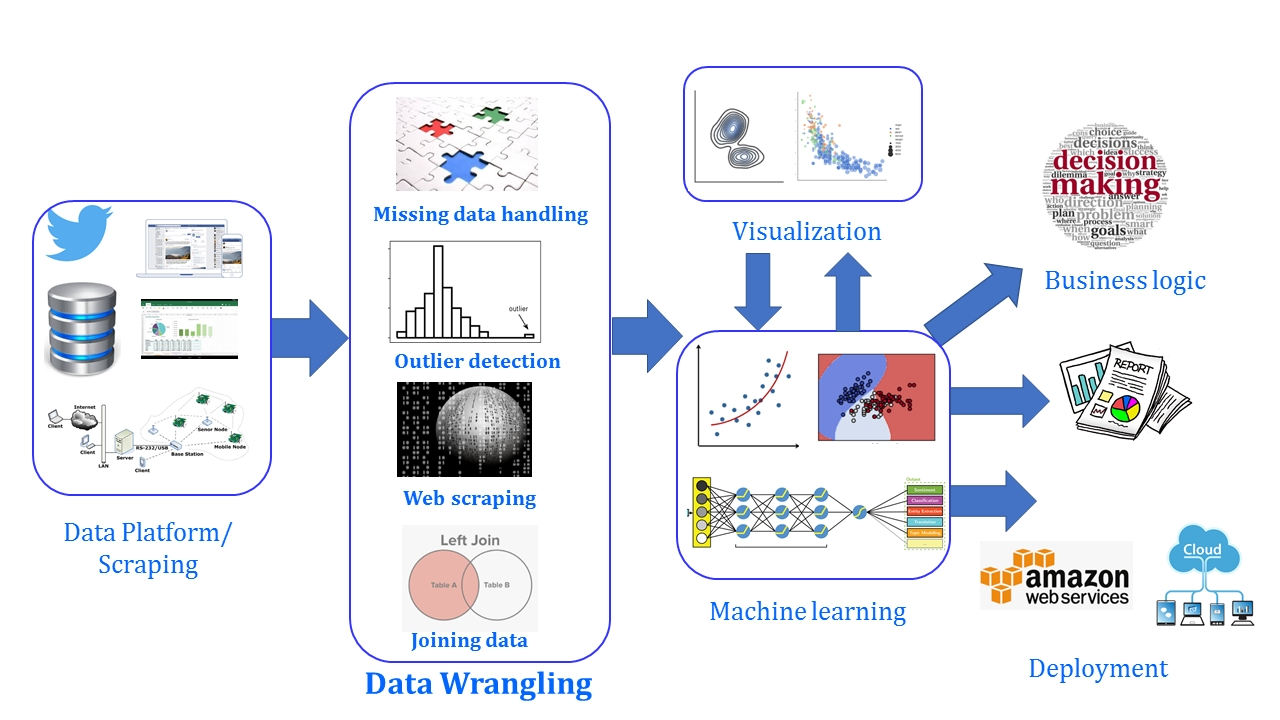Figure 1.1: Process of data wrangling

The process of data wrangling includes first finding the appropriate data that's necessary for the analysis. This data can be from one or multiple sources, such as tweets, bank transaction statements in a relational database, sensor data, and so on. This data needs to be cleaned. If there is missing data, we will either delete or substitute it, with the help of several techniques. If there are outliers, we need to first detect them and then handle them appropriately. If data is from multiple sources, we will have to perform join operations to combine it.

In an extremely rare situation, data wrangling may not be needed. For example, if the data that's necessary for a machine learning task is already stored in an acceptable format in an in-house database, then a simple SQL query may be enough to extract the data into a table, ready to be passed on to the modeling stage.

## Python for Data Wrangling

There is always a debate on whether to perform the wrangling process using an enterprise tool or by using a programming language and associated frameworks. There are many commercial, enterprise-level tools for data formatting and pre-processing that do not involve much coding on the part of the user. These examples include the following:

• General purpose data analysis platforms such as Microsoft Excel (with add-ins)

• Statistical discovery package such as JMP (from SAS)

• Modeling platforms such as RapidMiner

• Analytics platforms from niche players focusing on data wrangling, such as Trifacta, Paxata, and Alteryx

However, programming languages such as Python provide more flexibility, control, and power compared to these off-the-shelf tools.

As the volume, velocity, and variety (the three Vs of big data) of data undergo rapid changes, it is always a good idea to develop and nurture a significant amount of in-house expertise in data wrangling using fundamental programming frameworks so that an organization is not beholden to the whims and fancies of any enterprise platform for as basic a task as data wrangling: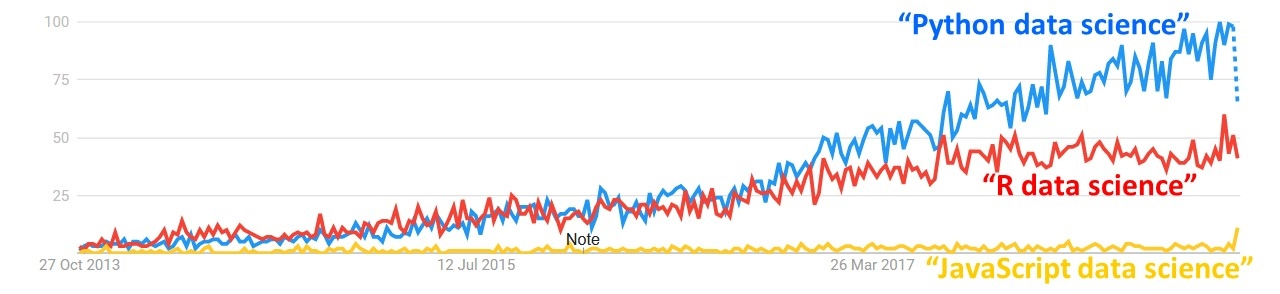Figure 1.2: Google trend worldwide over the last Five years

A few of the obvious advantages of using an open source, free programming paradigm such as Python for data wrangling are the following:

• General purpose open source paradigm putting no restriction on any of the methods you can develop for the specific problem at hand

• Great ecosystem of fast, optimized, open source libraries, focused on data analytics

• Growing support to connect Python to every conceivable data source type

• Easy interface to basic statistical testing and quick visualization libraries to check data quality

• Seamless interface of the data wrangling output with advanced machine learning models

Python is the most popular language of choice of machine learning and artificial intelligence these days.

## Lists, Sets, Strings, Tuples, and Dictionaries

Now that we have learned the importance of Python, we will start by exploring various basic data structures in Python. We will learn techniques to handle data. This is invaluable for a data practitioner.

We can issue the following command to start a new Jupyter server by typing the following in to the Command Prompt window:

`docker run -p 8888:8888 -v 'pwd':/notebooks -it rcshubhadeep/packt-data-wrangling-base:latest ipython`

This will start a jupyter server and you can visit it at http://localhost:8888 and use the passcode dw_4_all to access the main interface.

### Lists

Lists are fundamental Python data structures that have continuous memory locations, can host different data types, and can be accessed by the index.

We will start with a list and list comprehension. We will generate a list of numbers, and then examine which ones among them are even. We will sort, reverse, and check for duplicates. We will also see how many different ways we can access the list elements, iterating over them and checking the membership of an element.

The following is an example of a simple list:

`list_example = [51, 27, 34, 46, 90, 45, -19]`

The following is also an example of a list:

`list_example2 = [15, "Yellow car", True, 9.456, [12, "Hello"]]`

As you can see, a list can contain any number of the allowed datatype, such as int, float, string, and Boolean, and a list can also be a mix of different data types (including nested lists).

If you are coming from a strongly typed language, such as C, C++, or Java, then this will probably be strange as you are not allowed to mix different kinds of data types in a single array in those languages. Lists are somewhat like arrays, in the sense that they are both based on continuous memory locations and can be accessed using indexes. But the power of Python lists come from the fact that they can host different data types and you are allowed to manipulate the data.

### Note

Be careful, though, as the very power of lists, and the fact that you can mix different data types in a single list, can actually create subtle bugs that can be very difficult to track.

### Exercise 1: Accessing the List Members

In the following exercise, we will be creating a list and then observing the different ways of accessing the elements:

1. Define a list called list_1 with four integer members, using the following command:

`list_1 = [34, 12, 89, 1]`

The indices will be automatically assigned, as follows: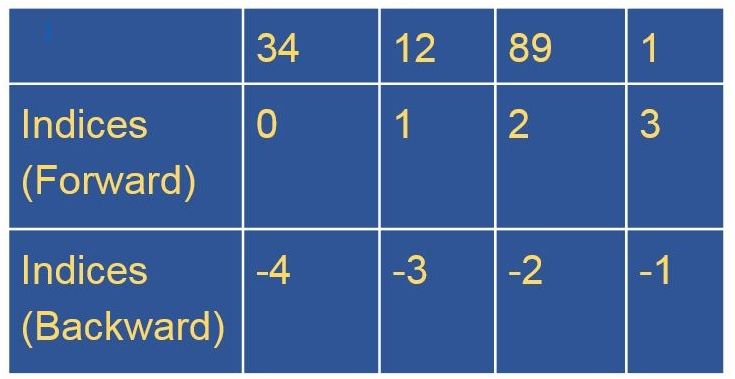Figure 1.3: List showing the forward and backward indices

2. Access the first element from list_1 using its forward index:

`list_1 #34`
3. Access the last element from list_1 using its forward index:

`list_1 #1`
4. Access the last element from list_1 using the len function:

`list_1[len(list_1) - 1] #1`

The len function in Python returns the length of the specified list.

5. Access the last element from list_1 using its backward index:

`list_1[-1] #1`
6. Access the first three elements from list_1 using forward indices:

`list_1[1:3] # [12, 89]`

This is also called list slicing, as it returns a smaller list from the original list by extracting only, a part of it. To slice a list, we need two integers. The first integer will denote the start of the slice and the second integer will denote the end-1 element.

### Note

Notice that slicing did not include the third index or the end element. This is how list slicing works.

1. Access the last two elements from list_1 by slicing:

`list_1[-2:] # [89, 1]`
2. Access the first two elements using backward indices:

`list_1[:-2] # [34, 12]`

When we leave one side of the colon (:) blank, we are basically telling Python either to go until the end or start from the beginning of the list. It will automatically apply the rule of list slices that we just learned.

3. Reverse the elements in the string:

`list_1[-1::-1] # [1, 89, 12, 34]`

### Note

The last bit of code is not very readable, meaning it is not obvious just by looking at it what it is doing. It is against Python's philosophy. So, although this kind of code may look clever, we should resist the temptation to write code like this.

### Exercise 2: Generating a List

We are going to examine various ways of generating a list:

1. Create a list using the append method:

```list_1 = []
for x in range(0, 10):
list_1.append(x)
list_1```

The output will be as follows:

`[0, 1, 2, 3, 4, 5, 6, 7, 8, 9]`

Here, we started by declaring an empty list and then we used a for loop to append values to it. The append method is a method that's given to us by the Python list data type.

2. Generate a list using the following command:

```list_2 = [x for x in range(0, 100)]
list_2```

The partial output is as follows: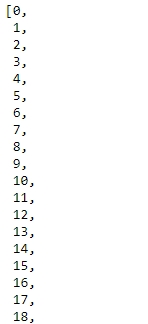Figure 1.4: List comprehension

This is list comprehension, which is a very powerful tool that we need to master. The power of list comprehension comes from the fact that we can use conditionals inside the comprehension itself.

3. Use a while loop to iterate over a list to understand the difference between a while loop and a for loop:

```i = 0
while i < len(list_1) :
print(list_1[i])
i += 1```

The partial output will be as follows: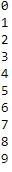Figure 1.5: Output showing the contents of list_1 using a while loop

4. Create list_3 with numbers that are divisible by 5:

```list_3 = [x for x in range(0, 100) if x % 5 == 0]
list_3```

The output will be a list of numbers up to 100 in increments of 5:

`[0, 5, 10, 15, 20, 25, 30, 35, 40, 45, 50, 55, 60, 65, 70, 75, 80, 85, 90, 95]`
5. Generate a list by adding the two lists:

```list_1 = [1, 4, 56, -1]
list_2 = [1, 39, 245, -23, 0, 45]
list_3 = list_1 + list_2
list_3```

The output is as follows:

`[1, 4, 56, -1, 1, 39, 245, -23, 0, 45]`
6. Extend a string using the extend keyword:

```list_1.extend(list_2)
list_1```

The partial output is as follows: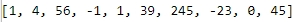Figure 1.6: Contents of list_1

The second operation changes the original list (list_1) and appends all the elements of list_2 to it. So, be careful when using it.

### Exercise 3: Iterating over a List and Checking Membership

We are going to iterate over a list and test whether a certain value exists in it:

1. Iterate over a list:

```list_1 = [x for x in range(0, 100)]
for i in range(0, len(list_1)):
print(list_1[i])```

The output is as follows: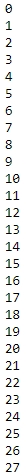Figure 1.7: Section of list_1

2. However, it is not very Pythonic. Being Pythonic is to follow and conform to a set of best practices and conventions that have been created over the years by thousands of very able developers, which in this case means to use the in keyword, because Python does not have index initialization, bounds checking, or index incrementing, unlike traditional languages. The Pythonic way of iterating over a list is as follows:

```for i in list_1:
print(i)```

The output is as follows: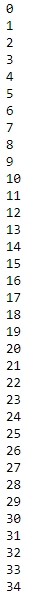Figure 1.8: A section of list_1

Notice that, in the second method, we do not need a counter anymore to access the list index; instead, Python's in operator gives us the element at the i th position directly.

3. Check whether the integers 25 and -45 are in the list using the in operator:

` 25 in list_1`

The output is True.

` -45 in list_1`

The output is False.

### Exercise 4: Sorting a List

We generated a list called list_1 in the previous exercise. We are going to sort it now:

### Note

The difference between the sort function and the reverse function is the fact that we can use sort with custom sorting functions to do custom sorting, whereas we can only use reverse to reverse a list. Here also, both the functions work in-place, so be aware of this while using them.

1. As the list was originally a list of numbers from 0 to 99, we will sort it in the reverse direction. To do that, we will use the sort method with reverse=True:

```list_1.sort(reverse=True)
list_1```

The partial output is as follows: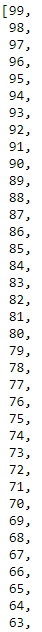Figure 1.9: Section of output showing the reversed list

2. We can use the reverse method directly to achieve this result:

```list_1.reverse()
list_1```

The output is as follows: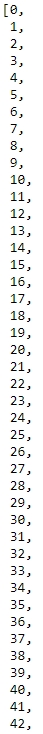Figure 1.10: Section of output after reversing the string

### Exercise 5: Generating a Random List

In this exercise, we will be generating a list with random numbers:

1. Import the random library:

`import random`
2. Use the randint function to generate random integers and add them to a list:

`list_1 = [random.randint(0, 30) for x in range (0, 100)]`
3. Print the list using print(list_1). Note that there will be duplicate values in list_1:

`list_1`

The sample output is as follows: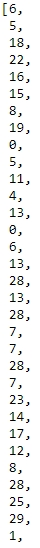Figure 1.11: Section of the sample output for list_1

There are many ways to get a list of unique numbers, and while you may be able to write a few lines of code using a for loop and another list (you should actually try doing it!), let's see how we can do this without a for loop and with a single line of code. This will bring us to the next data structure, sets.

### Activity 1: Handling Lists

In this activity, we will generate a list of random numbers and then generate another list from the first one, which only contains numbers that are divisible by three. Repeat the experiment three times. Then, we will calculate the average difference of length between the two lists.

These are the steps for completing this activity:

1. Create a list of 100 random numbers.

2. Create a new list from this random list, with numbers that are divisible by 3.

3. Calculate the length of these two lists and store the difference in a new variable.

4. Using a loop, perform steps 2 and 3 and find the difference variable three times.

5. Find the arithmetic mean of these three difference values.

### Note

The solution for this activity can be found on page 282.

### Sets

A set, mathematically speaking, is just a collection of well-defined distinct objects. Python gives us a straightforward way to deal with them using its set datatype.

### Introduction to Sets

With the last list that we generated, we are going to revisit the problem of getting rid of duplicates from it. We can achieve that with the following line of code:

`list_12 = list(set(list_1))`

If we print this, we will see that it only contains unique numbers. We used the set data type to turn the first list into a set, thus getting rid of all duplicate elements, and then we used the list function on it to turn it into a list from a set once more:

`list_12`

The output will be as follows: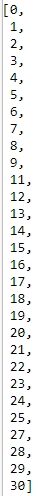Figure 1.12: Section of output for list_21

### Union and Intersection of Sets

This is what a union between two sets looks like: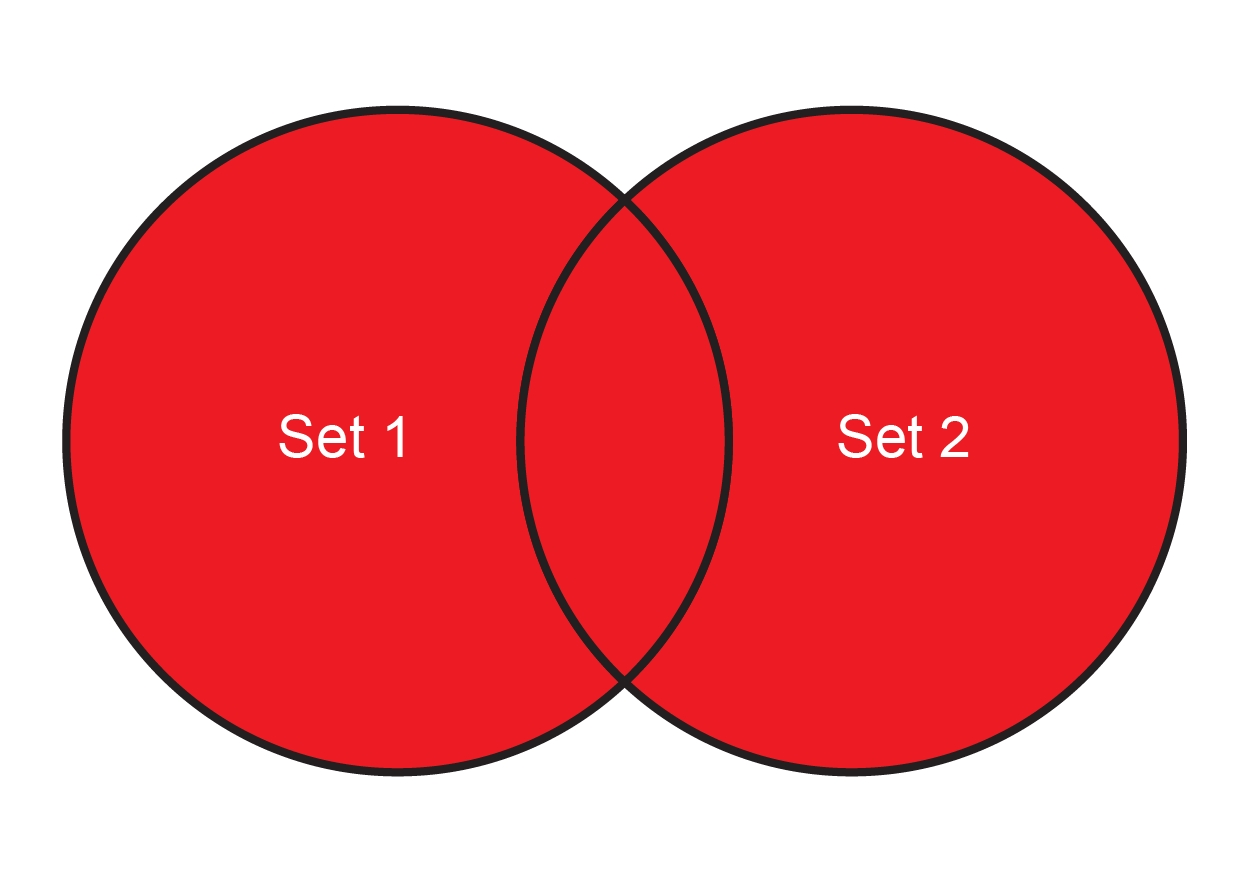Figure 1.13: Venn diagram showing the union of two sets

This simply means take everything from both sets but take the common elements only once.

We can create this using the following code:

```set1 = {"Apple", "Orange", "Banana"}
set2 = {"Pear", "Peach", "Mango", "Banana"}```

To find the union of the two sets, the following instructions should be used:

`set1 | set2`

The output would be as follows:

`{'Apple', 'Banana', 'Mango', 'Orange', 'Peach', 'Pear'}`

Notice that the common element, Banana, appears only once in the resulting set. The common elements between two sets can be identified by obtaining the intersection of the two sets, as follows: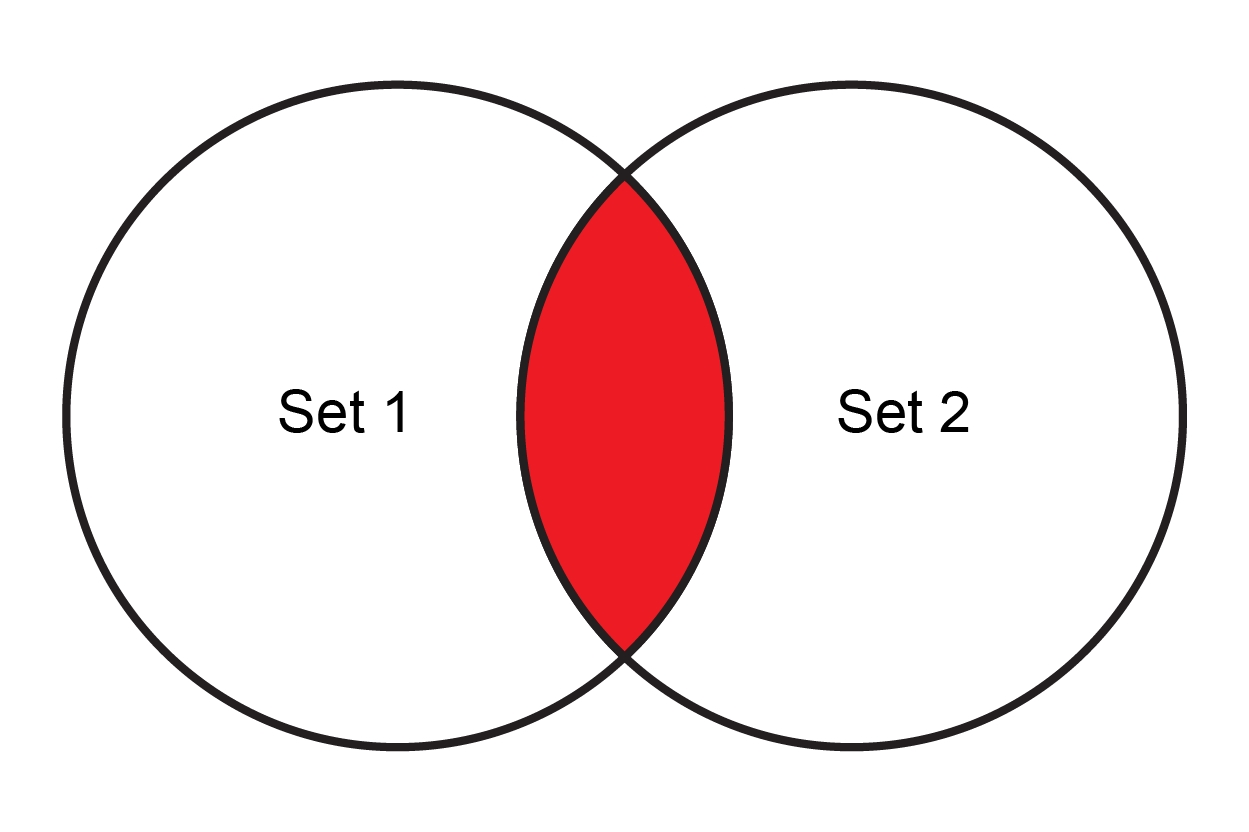Figure 1.14: Venn diagram showing the intersection of two sets

We get the intersection of two sets in Python as follows:

`set1 & set2`

This will give us a set with only one element. The output is as follows:

`{'Banana'}`

### Note

You can also calculate the difference between sets (also known as complements). To find out more, refer to this link: https://docs.python.org/3/tutorial/datastructures.html#sets.

### Creating Null Sets

You can create a null set by creating a set containing no elements. You can do this by using the following code:

```null_set_1 = set({})
null_set_1```

The output is as follows:

`set()`

However, to create a dictionary, use the following command:

```null_set_2 = {}
null_set_2```

The output is as follows:

`{}`

### Dictionary

A dictionary is like a list, which means it is a collection of several elements. However, with the dictionary, it is a collection of key-value pairs, where the key can be anything that can be hashed. Generally, we use numbers or strings as keys.

To create a dictionary, use the following code:

```dict_1 = {"key1": "value1", "key2": "value2"}
dict_1```

The output is as follows:

`{'key1': 'value1', 'key2': 'value2'}`

This is also a valid dictionary:

```dict_2 = {"key1": 1, "key2": ["list_element1", 34], "key3": "value3",
"key4": {"subkey1": "v1"}, "key5": 4.5}
dict_2```

The output is as follows:

```{'key1': 1,
'key2': ['list_element1', 34],
'key3': 'value3',
'key4': {'subkey1': 'v1'},
'key5': 4.5}```

The keys must be unique in a dictionary.

### Exercise 6: Accessing and Setting Values in a Dictionary

In this exercise, we are going to access and set values in a dictionary:

1. Access a particular key in a dictionary:

`dict_2["key2"]`

This will return the value associated with it as follows:

`['list_element1', 34]`
2. Assign a new value to the key:

`dict_2["key2"] = "My new value"`
3. Define a blank dictionary and then use the key notation to assign values to it:

```dict_3 = {}  # Not a null set. It is a dict
dict_3["key1"] = "Value1"
dict_3```

The output is as follows:

`{'key1': 'Value1'}`

### Exercise 7: Iterating Over a Dictionary

In this exercise, we are going to iterate over a dictionary:

1. Create dict_1:

`dict_1 = {"key1": 1, "key2": ["list_element1", 34], "key3": "value3", "key4": {"subkey1": "v1"}, "key5": 4.5}`
2. Use the looping variables k and v:

```for k, v in dict_1.items():
print("{} - {}".format(k, v))```

The output is as follows:

```key1 - 1
key2 - ['list_element1', 34]
key3 - value3
key4 - {'subkey1': 'v1'}
key5 - 4.5```

### Note

Notice the difference between how we did the iteration on the list and how we are doing it here.

### Exercise 8: Revisiting the Unique Valued List Problem

We will use the fact that dictionary keys cannot be duplicated to generate the unique valued list:

1. First, generate a random list with duplicate values:

`list_1 = [random.randint(0, 30) for x in range (0, 100)]`
2. Create a unique valued list from list_1:

`list(dict.fromkeys(list_1).keys())`

The sample output is as follows: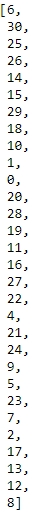Figure 1.15: Output showing the unique valued list

Here, we have used two useful functions on the dict data type in Python, fromkeys and keys. fromkeys creates a dict where the keys come from the iterable (in this case, which is a list), values default to None, and keys give us the keys of a dict.

### Exercise 9: Deleting Value from Dict

In this exercise, we are going to delete a value from a dict:

1. Create list_1 with five elements:

```dict_1 = {"key1": 1, "key2": ["list_element1", 34], "key3": "value3",
"key4": {"subkey1": "v1"}, "key5": 4.5}
dict_1```

The output is as follows:

```{'key1': 1,
'key2': ['list_element', 34],
'key3': 'value3',
'key4': {'subkey1': 'v1'},
'key5': 4.5}```
2. We will use the del function and specify the element:

`del dict_1["key2"]`

The output is as follows:

`{'key3': 'value3', 'key4': {'subkey1': 'v1'}, 'key5': 4.5}`

### Note

The del operator can be used to delete a specific index from a list as well.

### Exercise 10: Dictionary Comprehension

In this final exercise on dict, we will go over a less used comprehension than the list one: dictionary comprehension. We will also examine two other ways to create a dict, which will be useful in the future.

A dictionary comprehension works exactly the same way as the list one, but we need to specify both the keys and values:

1. Generate a dict that has 0 to 9 as the keys and the square of the key as the values:

```list_1 = [x for x in range(0, 10)]
dict_1 = {x : x**2 for x in list_1}
dict_1```

The output is as follows:

`{0: 0, 1: 1, 2: 4, 3: 9, 4: 16, 5: 25, 6: 36, 7: 49, 8: 64, 9: 81}`

Can you generate a dict using dict comprehension where the keys are from 0 to 9 and the values are the square root of the keys? This time, we won't use a list.

2. Generate a dictionary using the dict function:

```dict_2 = dict([('Tom', 100), ('Dick', 200), ('Harry', 300)])
dict_2```

The output is as follows:

`{'Tom': 100, 'Dick': 200, 'Harry': 300}`

You can also generate dictionary using the dict function, as follows:

```dict_3 = dict(Tom=100, Dick=200, Harry=300)
dict_3```

The output is as follows:

`{'Tom': 100, 'Dick': 200, 'Harry': 300}`

It is pretty versatile. So, both the preceding commands will generate valid dictionaries.

The strange looking pair of values that we had just noticed ('Harry', 300) is called a tuple. This is another important fundamental data type in Python. We will learn about tuples in the next topic.

### Tuples

A tuple is another data type in Python. It is sequential in nature and similar to lists.

A tuple consists of values separated by commas, as follows:

`tuple_1 = 24, 42, 2.3456, "Hello"`

Notice that, unlike lists, we did not open and close square brackets here.

### Creating a Tuple with Different Cardinalities

This is how we create an empty tuple:

`tuple_1 = ()`

And this is how we create a tuple with only one value:

`tuple_1 = "Hello",`

Notice the trailing comma here.

We can nest tuples, similar to list and dicts, as follows:

```tuple_1 = "hello", "there"
tuple_12 = tuple_1, 45, "Sam"```

One special thing about tuples is the fact that they are an immutable data type. So, once created, we cannot change their values. We can just access them, as follows:

```tuple_1 = "Hello", "World!"
tuple_1 = "Universe!"```

The last line of code will result in a TypeError as a tuple does not allow modification.

This makes the use case for tuples a bit different than lists, although they look and behave very similarly in a few aspects.

### Unpacking a Tuple

The term unpacking a tuple simply means to get the values contained in the tuple in different variables:

```tuple_1 = "Hello", "World"
hello, world = tuple_1
print(hello)
print(world)```

The output is as follows:

```Hello
World```

Of course, as soon as we do that, we can modify the values contained in those variables.

### Exercise 11: Handling Tuples

1. Create a tuple to demonstrate how tuples are immutable. Unpack it to read all elements, as follows:

```tupleE = "1", "3", "5"
tupleE```

The output is as follows:

`('1', '3', '5')`
2. Try to override a variable from the tupleE tuple:

`tupleE = "5"`

This step will result in TypeError as the tuple does not allow modification.

3. Try to assign a series to the tupleE tuple:

`1, 3, 5 = tupleE`
4. Print the output:

```print(1)
print(3)```

The output is as follows:

```1
3```

We have mainly seen two different types of data so far. One is represented by numbers; another is represented by textual data. Whereas numbers have their own tricks, which we will see later, it is time to look into textual data in a bit more detail.

### Strings

In the final section of this section, we will learn about strings. Strings in Python are similar to any other programming language.

This is a string:

`string1 = 'Hello World!' `

A string can also be declared in this manner:

`string2 = "Hello World 2!"`

You can use single quotes and double quotes to define a string.

### Exercise 12: Accessing Strings

Strings in Python behave similar to lists, apart from one big caveat. Strings are immutable, whereas lists are mutable data structures:

1. Create a string called str_1:

`str_1 = "Hello World!"`

Access the elements of the string by specifying the location of the element, like we did in lists.

2. Access the first member of the string:

`str_1`

The output is as follows:

`'H'`
3. Access the fourth member of the string:

`str_1`

The output is as follows:

`'o'`
4. Access the last member of the string:

`str_1[len(str_1) - 1]`

The output is as follows:

`'!'`
5. Access the last member of the string:

`str_1[-1]`

The output is as follows:

`'!'`

Each of the preceding operations will give you the character at the specific index.

### Note

The method for accessing the elements of a string is like accessing a list.

### Exercise 13: String Slices

Just like lists, we can slice strings:

1. Create a string, str_1:

`str_1 = "Hello World! I am learning data wrangling"`
2. Specify the slicing values and slice the string:

`str_1[2:10]`

The output is this:

'llo Worl'

3. Slice a string by skipping a slice value:

`str_1[-31:]`

The output is as follows:

`'d! I am learning data wrangling'`
4. Use negative numbers to slice the string:

`str_1[-10:-5]`

The output is as follows:

`' wran'`

### String Functions

To find out the length of a string, we simply use the len function:

```str_1 = "Hello World! I am learning data wrangling"
len(str_1)```

The length of the string is 41. To convert a string's case, we can use the lower and upper methods:

```str_1 = "A COMPLETE UPPER CASE STRING"
str_1.lower()
str_1.upper()```

The output is as follows:

`'A COMPLETE UPPER CASE STRING'`

To search for a string within a string, we can use the find method:

```str_1 = "A complicated string looks like this"
str_1.find("complicated")
str_1.find("hello")# This will return -1```

The output is -1. Can you figure out whether the find method is case-sensitive or not? Also, what do you think the find method returns when it actually finds the string?

To replace one string with another, we have the replace method. Since we know that a string is an immutable data structure, replace actually returns a new string instead of replacing and returning the actual one:

```str_1 = "A complicated string looks like this"
str_1.replace("complicated", "simple")```

The output is as follows:

`'A simple string looks like this'`

You should look up string methods in the standard documentation of Python 3 to discover more about these methods.

### Exercise 14: Split and Join

These two string methods need separate introductions, as they enable you to convert a string into a list and vice versa:

1. Create a string and convert it to a list using the split method:

```str_1 = "Name, Age, Sex, Address"
list_1 = str_1.split(",")
list_1```

The preceding code will give you a list similar to the following:

`['Name', ' Age', ' Sex', ' Address']`
2. Combine this list into another string using the join method:

`" | ".join(list_1) `

This code will give you a string like this:

`'Name |  Age |  Sex |  Address'`

With these, we are at the end of our second topic of this chapter. We now have the motivation to learn data wrangling and have a solid introduction to the fundamentals of data structures using Python. There is more to this topic, which will be covered in a future chapters.

We have designed an activity for you so that you can practice all the skills you just learned. This small activity should take around 30 to 45 minutes to finish.

### Activity 2: Analyze a Multiline String and Generate the Unique Word Count

This section will ensure that you have understood the various basic data structures and their manipulation. We will do that by going through an activity that has been designed specifically for this purpose.

In this activity, we will do the following:

• Get multiline text and save it in a Python variable

• Get rid of all new lines in it using string methods

• Get all the unique words and their occurrences from the string

• Repeat the step to find all unique words and occurrences, without considering case sensitivity

### Note

For the sake of simplicity for this activity, the original text (which can be found at https://www.gutenberg.org/files/1342/1342-h/1342-h.htm) has been pre-processed a bit.

These are the steps to guide you through solving this activity:

1. Create a mutliline_text variable by copying the text from the first chapter of Pride and Prejudice.

### Note

The first chapter of Pride and Prejudice by Jane Austen has been made available on the GitHub repository at https://github.com/TrainingByPackt/Data-Wrangling-with-Python/blob/master/Chapter01/Activity02/.

2. Find the type and length of the multiline_text string using the commands type and len.

3. Remove all new lines and symbols using the replace function.

4. Find all of the words in multiline_text using the split function.

5. Create a list from this list that will contain only the unique words.

6. Count the number of times the unique word has appeared in the list using the key and value in dict.

7. Find the top 25 words from the unique words that you have found using the slice function.

You just created, step by step, a unique word counter using all the neat tricks that you learned about in this chapter.

### Note

The solution for this activity can be found on page 285.

## Summary

In this chapter, we learned what the term data wrangling means. We also got examples from various real-life data science situations where data wrangling is very useful and is used in industry. We moved on to learn about the different built-in data structures that Python has to offer. We got our hands dirty by exploring lists, sets, dictionaries, tuples, and strings. They are the fundamental building blocks in Python data structures, and we need them all the time while working and manipulating data in Python. We did several small hands-on exercises to learn more about them. We finished this chapter with a carefully designed activity, which let us combine a lot of different tricks from all the different data structures into a real-life situation and let us observe the interplay between all of them.

In the next chapter, we will learn about the data structures in Python and utilize them to solve real-world problems.

• ##### Dr. Tirthajyoti Sarkar

Dr. Tirthajyoti Sarkar works as a senior principal engineer in the semiconductor technology domain, where he applies cutting-edge data science/machine learning techniques for design automation and predictive analytics. He writes regularly about Python programming and data science topics. He holds a Ph.D. from the University of Illinois and certifications in artificial intelligence and machine learning from Stanford and MIT.

Browse publications by this author